Anúncio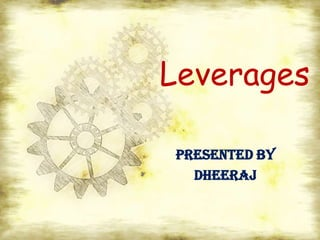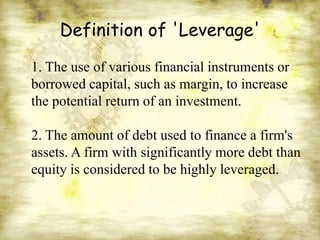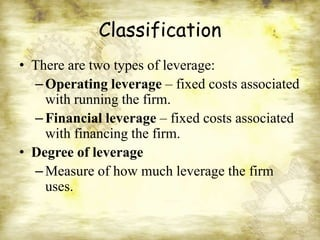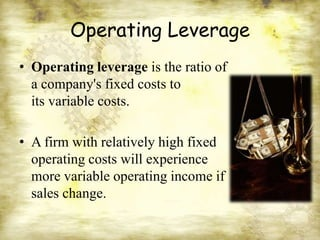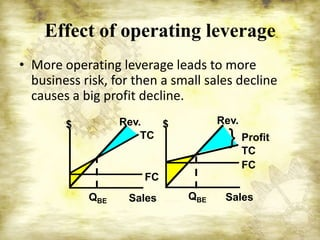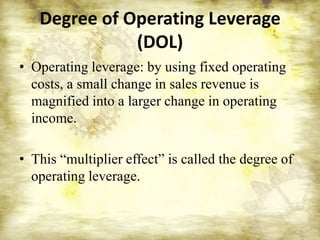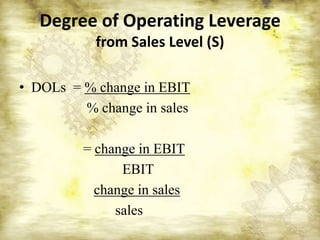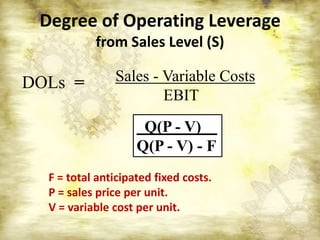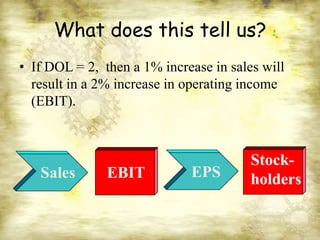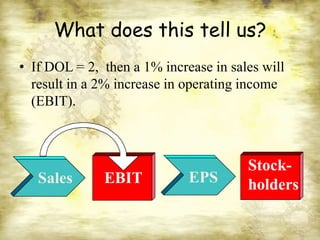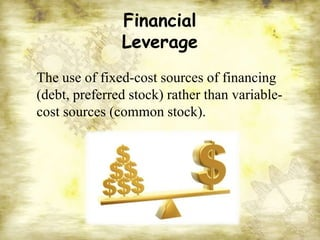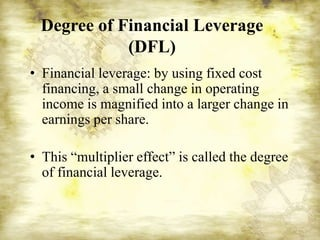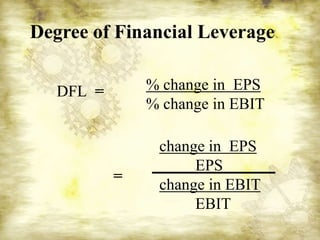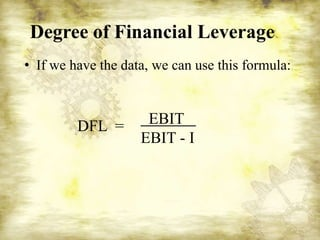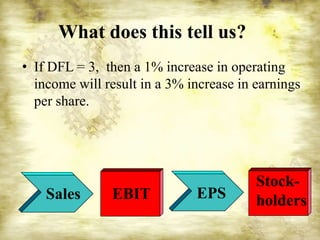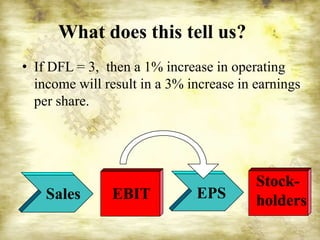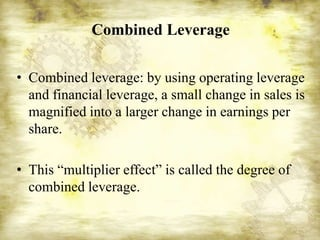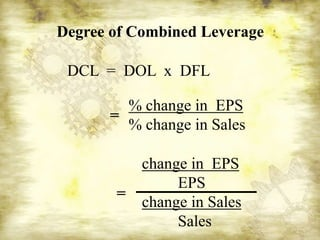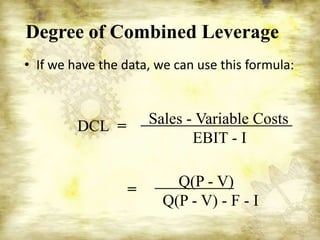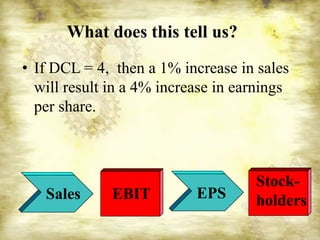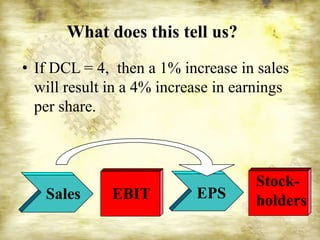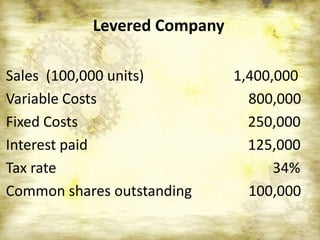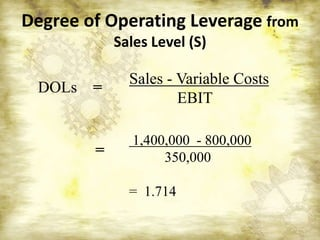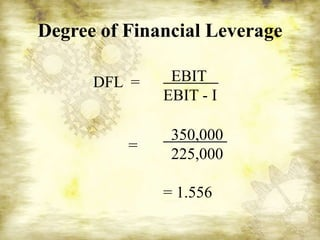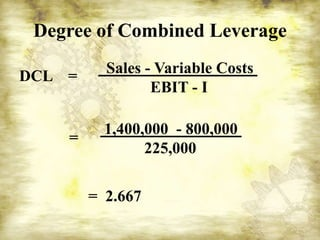1 de 26
Anúncio

### Leverages

1. Leverages PRESENTED BY DHEERAJ
2. Definition of 'Leverage' 1. The use of various financial instruments or borrowed capital, such as margin, to increase the potential return of an investment. 2. The amount of debt used to finance a firm's assets. A firm with significantly more debt than equity is considered to be highly leveraged.
3. Classification • There are two types of leverage: – Operating leverage – fixed costs associated with running the firm. – Financial leverage – fixed costs associated with financing the firm. • Degree of leverage – Measure of how much leverage the firm uses.
4. Operating Leverage • Operating leverage is the ratio of a company's fixed costs to its variable costs. • A firm with relatively high fixed operating costs will experience more variable operating income if sales change.
5. Effect of operating leverage • More operating leverage leads to more business risk, for then a small sales decline causes a big profit decline. \$ Rev. \$ Rev. TC } Profit TC FC FC QBE Sales QBE Sales
6. Degree of Operating Leverage (DOL) • Operating leverage: by using fixed operating costs, a small change in sales revenue is magnified into a larger change in operating income. • This “multiplier effect” is called the degree of operating leverage.
7. Degree of Operating Leverage from Sales Level (S) • DOLs = % change in EBIT % change in sales = change in EBIT EBIT change in sales sales
8. Degree of Operating Leverage from Sales Level (S) DOLs = Sales - Variable Costs EBIT Q(P - V) Q(P - V) - F F = total anticipated fixed costs. P = sales price per unit. V = variable cost per unit.
9. What does this tell us? • If DOL = 2, then a 1% increase in sales will result in a 2% increase in operating income (EBIT). Stock- Sales EBIT EPS holders
10. What does this tell us? • If DOL = 2, then a 1% increase in sales will result in a 2% increase in operating income (EBIT). Stock- Sales EBIT EPS holders
11. Financial Leverage The use of fixed-cost sources of financing (debt, preferred stock) rather than variable- cost sources (common stock).
12. Degree of Financial Leverage (DFL) • Financial leverage: by using fixed cost financing, a small change in operating income is magnified into a larger change in earnings per share. • This “multiplier effect” is called the degree of financial leverage.
13. Degree of Financial Leverage DFL = % change in EPS % change in EBIT change in EPS EPS = change in EBIT EBIT
14. Degree of Financial Leverage • If we have the data, we can use this formula: DFL = EBIT EBIT - I
15. What does this tell us? • If DFL = 3, then a 1% increase in operating income will result in a 3% increase in earnings per share. Stock- Sales EBIT EPS holders
16. What does this tell us? • If DFL = 3, then a 1% increase in operating income will result in a 3% increase in earnings per share. Stock- Sales EBIT EPS holders
17. Combined Leverage • Combined leverage: by using operating leverage and financial leverage, a small change in sales is magnified into a larger change in earnings per share. • This “multiplier effect” is called the degree of combined leverage.
18. Degree of Combined Leverage DCL = DOL x DFL % change in EPS = % change in Sales change in EPS EPS = change in Sales Sales
19. Degree of Combined Leverage • If we have the data, we can use this formula: DCL = Sales - Variable Costs EBIT - I Q(P - V) = Q(P - V) - F - I
20. What does this tell us? • If DCL = 4, then a 1% increase in sales will result in a 4% increase in earnings per share. Stock- Sales EBIT EPS holders
21. What does this tell us? • If DCL = 4, then a 1% increase in sales will result in a 4% increase in earnings per share. Stock- Sales EBIT EPS holders
22. Levered Company Sales (100,000 units) 1,400,000 Variable Costs 800,000 Fixed Costs 250,000 Interest paid 125,000 Tax rate 34% Common shares outstanding 100,000
23. Degree of Operating Leverage from Sales Level (S) Sales - Variable Costs DOLs = EBIT 1,400,000 - 800,000 = 350,000 = 1.714
24. Degree of Financial Leverage DFL = EBIT EBIT - I 350,000 = 225,000 = 1.556
25. Degree of Combined Leverage DCL = Sales - Variable Costs EBIT - I 1,400,000 - 800,000 = 225,000 = 2.667
Anúncio